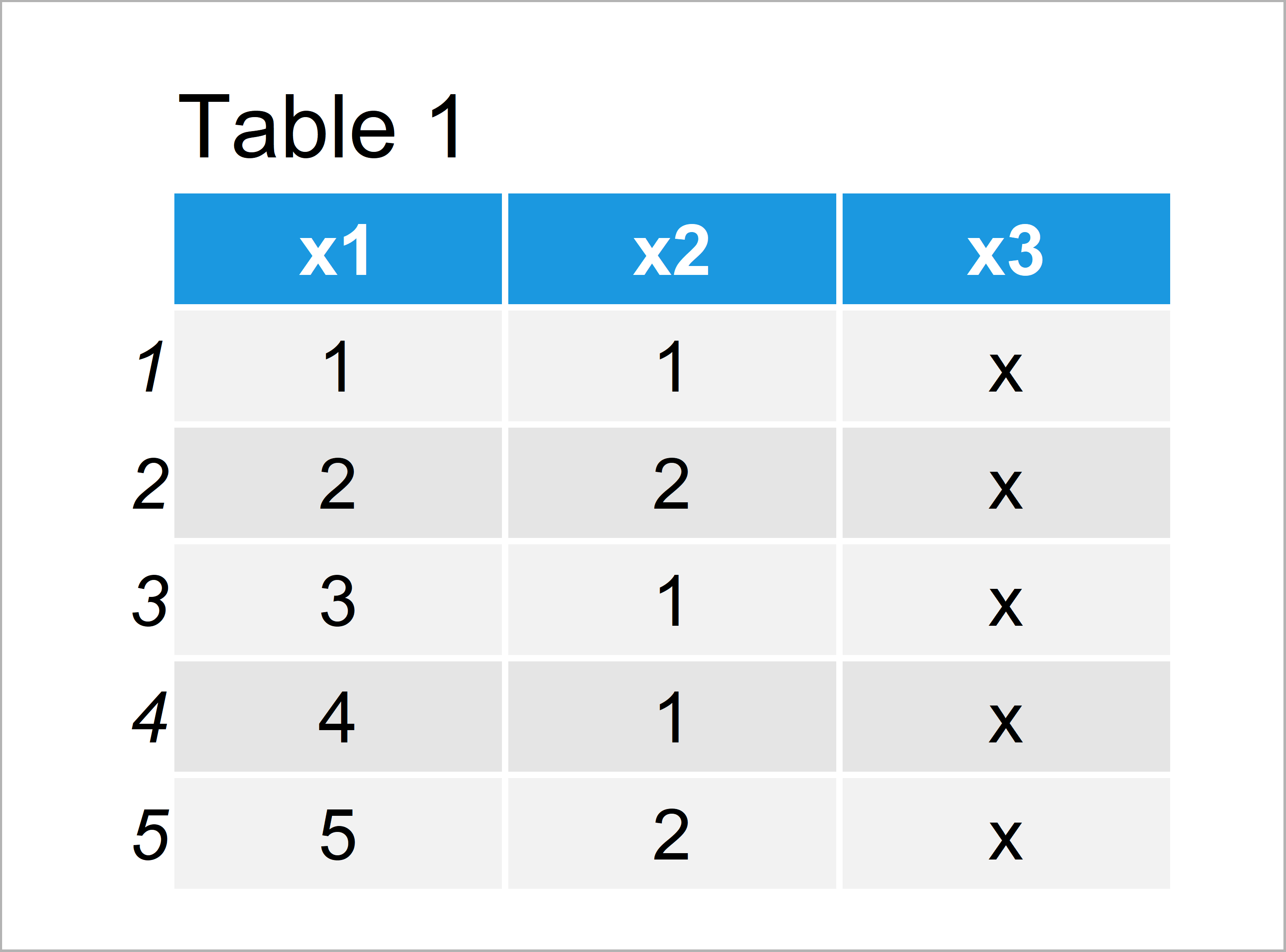# Extract & Count Unique Values in Each Column of Data Frame in R (2 Examples)

This tutorial explains how to get the unique value (counts) in all data frame columns in R.

The article is structured as follows:

Let’s do this.

## Creation of Example Data

Consider the following example data:

```data <- data.frame(x1 = 1:5,           # Create example data
x2 = c(1, 2, 1, 1, 2),
x3 = "x")
data                                   # Print example data```Have a look at the table that has been returned after executing the previous R programming syntax. It shows that our example data has five rows and three variables.

## Example 1: Create List Containing Unique Values of Each Column

In this example, I’ll show how to return a list that contains the unique values of each variable in our data frame.

To create such a list, we can use the lapply and unique functions as shown below:

```list_unique <- lapply(data, unique)    # List with unique values
list_unique                            # Print list
# \$x1
#  1 2 3 4 5
#
# \$x2
#  1 2
#
# \$x3
#  "x"
#```

Have a look at the previous output of the RStudio console: It shows a list containing one list element for each column of our data frame. Each of these list elements displays the unique values of the different columns.

## Example 2: Create Vector Containing Count of Unique Values of Each Column

This example shows how to count unique values by columns of a data frame.

For this, we can use the rapply, length, and unique functions as shown below:

```count_unique <- rapply(data,           # Counts of unique values
function(x) length(unique(x)))
count_unique                           # Print counts
# x1 x2 x3
#  5  2  1```

The previous output shows the counts of unique values for each column of our input data frame. The variable x1 contains five unique values, the variable x2 contains two unique values, and the variable x3 contains only one unique value.

## Video, Further Resources & Summary

In case you need further information on the contents of this tutorial, I recommend watching the following video of my YouTube channel. In the video, I’m explaining the contents of this article.

Please accept YouTube cookies to play this video. By accepting you will be accessing content from YouTube, a service provided by an external third party.If you accept this notice, your choice will be saved and the page will refresh.

Besides the video, you could read the other tutorials on this homepage.

You have learned in this article how to return the actual unique values and the number of unique values in each variable of a data frame in R programming. In case you have additional questions, let me know in the comments section. Furthermore, don’t forget to subscribe to my email newsletter in order to get updates on the newest tutorials.

Subscribe to the Statistics Globe Newsletter